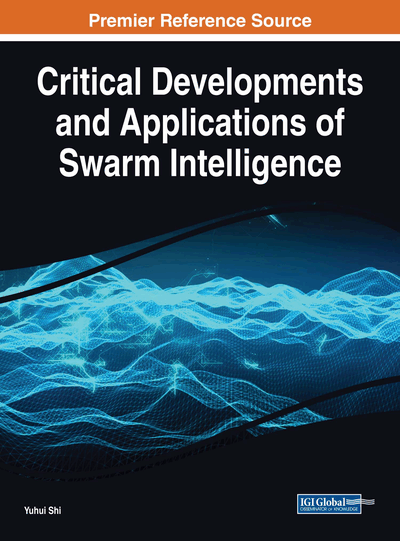# Multi-Thresholding of Histopathological Images Using Fuzzy Entropy and Parameterless Cuckoo Search

Krishna Gopal Gopal Dhal (Midnapore College (Autonomous), India), Mandira Sen (Tata Consultancy Services, India) and Sanjoy Das (University of Kalyani, India)
DOI: 10.4018/978-1-5225-5134-8.ch013
Available
\$37.50
No Current Special Offers

## Abstract

This chapter presents a multi-level histopathological image thresholding approach based on fuzzy entropy theory. This entropy measure is maximized to obtain the optimal thresholds of the image. In order to solve this problem, one self-adaptive and parameter-less cuckoo search (CS) algorithm has been employed, which leads to an accurate convergence towards the optima within less computational time. The performance of the proposed CS is also compared with traditional CS (TCS) algorithm and particle swarm optimization (PSO). The outcomes of the proposed fuzzy entropy-based model are compared with Shannon entropy-based model both visually and statistically in order to establish the perceptible difference in image.
Chapter Preview
Top

## Introduction

Image thresholding,which is the most significant and highly complicated task in low-level image analysis,is the process of extracting the objects from its background based on threshold levels. Several image segmentation processes, such as gray level thresholding, interactive pixel classification, neural network based approaches, edge detection and fuzzy based segmentation, etc.are reported in literature (Riseman, E.M., Arbib, M.A. (1977), Weszka, J.S. (1978), Fu, K.S., Mui, J.K. (1981), Haralharick, R.M., Shapiro, L.G. (1985), Borisenko, V.I., Zlatotol, A.A., Muchnik, I.B. (1987), Sahoo, P.K., Soltani, S., Wong, A.K.C., Chen, Y.C. (1988), Pal, N.R., Pal, S.K. (1993)).Global thresholding based segmentation for graylevel image is the well-known technique. Entropy based global thresholding techniques are reported in literature such as Kapur’s entropy (Kapur, J.N., Sahoo, P.K., Wong, A.K.C. (1985)), Sahoo et.al. (Wong, A.K.C., Sahoo, P.K. (1989)), Pun et. al. (T. Pun (1981)), Pal et.al.(Pal, N.R. (1996)), Li et.al.(Li, C.H., Lee, C.K. (1993)), Rosin et.al.(Rosin, P.L. (2001)), Reyni’s entropy (Sarkar S., Das S., Chaudhuri S. S., (2016.)), Tsallis entropy (Bhandari A. K., Kumar A., Singh G. K.(2015)). Multi-thresholding based image segmentation represents the splitting the image into different regions by selecting multiple threshold points. Otsu developed one multi-level image segmentation method based on region variance. Kapur et. al. proposed entropy based method for segmentation where the sum of the class entropy was maximized to find the optimal threshold (Kapur, J.N., Sahoo, P.K., Wong, A.K.C. (1985)). But the majority of the said techniques do not consider the inexactness of the gray levels i.e. if there is no clear boundary among different region leads to some ambiguity. In order to overcome the ambiguity problem, fuzzy theory is introduced in entropy based segmentation.Luca and Termini (Luca, A.D., Termini, S.(1972)) introduced a fuzzy partition technique for image segmentation.Bloch et. al. Employed fuzzy spatial relationship in image processing andimage interpretation area (Bloch, I.(2005)). Notably, application of fuzzy multi-level thresholding approach was performed by Zhao et. al. where three membership functions for 3-level thresholding i.e. dark, medium and bright was employed (Zhao, M.S., Fu, A.M.N., Yan, H.(2001)). Based on this paper, Tao et. al. developed one 3-level fuzzy entropy based segmentation technique by using Z-function, F-function and S-function as membership functions (Tao, W.B., Tian, J.W., Liu, J.(2003)). A thresholding approach based on the maximum fuzzy entropy principal over two dimensional histogram had been discussed by Cheng et. al. (H.D. Cheng, Y.H. Chen, and X.H Jiang.(2000)) where inter pixel relationship was taken into account. The threshold levels of the multi-thresholding problem were computed by employing metaheuristic algorithms as it helps to reduce the computational time (Olive, D., Cuevas, E., Pajares, G., Zaldivar, D., Perez-Cisneros, M.(2013), Krishna Priya,R., Thangaraj, C., Kesavadas, C., Kannan, S.(2013)). One Modified Particle Swarm Optimization (MPSO) was employed with fuzzy entropy in the MRI image segmentation field and the proposed model gave promising result in terms of visual analysis, statistical analysis and computational time (Krishna Priya,R., Thangaraj, C., Kesavadas, C., Kannan, S.(2013)). Sarkar et. al. proposed one Differential Evolution (DE) with fuzzy entropy based segmentation model which outperforms Shannon’s entropy based model visually and statistically (Sarkar, S., Paul, S., Burman, R., Das, S., Chaudhuri, S.S.(2015)). DE helped to find the optimal threshold values within less computational time. In this study, fuzzy entropy based multi-thresholding model is used to segment the histopathological images which is an untouched area as per best of our knowledge. One modified CS algorithm is employed for finding the fuzzy parameters and one comparative study among traditional CS, PSO and proposed CS algorithm has been performed in this study in terms of different statistical test and computational time. The fuzzy entropy based model is also compared with Shannon entropy based model. The visual and statistical results proved that the proposed model outperforms the Shannon entropy model in the histopathological image segmentation field. The chapter is composed of mainly four section. First section demonstrates the concept of multi-level fuzzy entropy. Second section represents the methodology behind the development of modified CS. In third section, discussion about the experimental results has been performed. The chapter is concluded in the last section.

## Complete Chapter List

Search this Book:
Reset